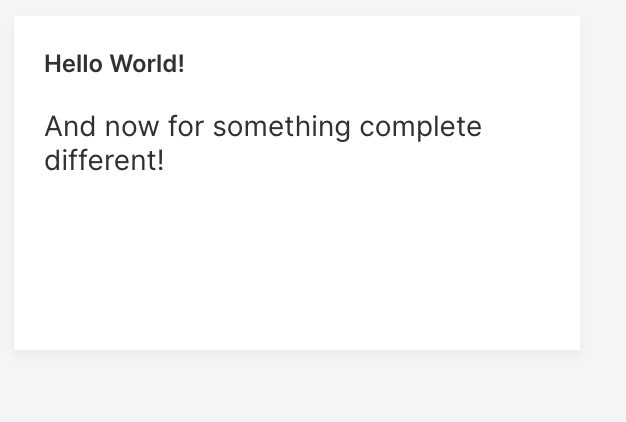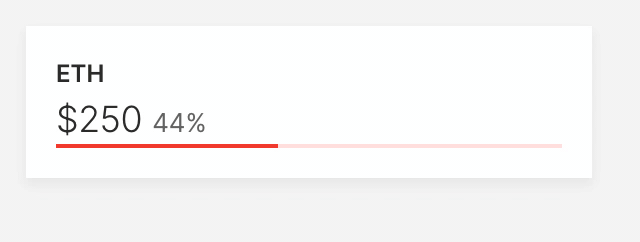# Introducing R API for Wave

Today, we are happy to announce preliminary support for the R language in H2O Wave. We now ship an R package that allows you build and control dashboards in Wave, and has feature-parity with Python Wave Scripts.

## Introduction​

The R language driver allows you to author R programs that can publish and control live web content using the Wave server. The entire suite of cards, components and page layout controls previously only available via Python are now accessible via R.

H2O Wave supports two kinds of programs:

• Wave apps are interactive programs that can update content and respond to user actions.
• Wave scripts are simpler, non-interactive (batch) programs: they can update content, but not respond to user actions.

The current release of the R language driver only supports Wave scripts. Support for Wave apps will ship in the near future.

## Installation​

1. Install the prerequisites:
``> install.packages(c("R6","devtools","jsonlite","stringr","httr"))``

2. There are two ways to install `h2owave`

From command line:

``\$ R CMD INSTALL h2owave_0.0.14.tar.gz``

From R interactive environment:

``> install.packages(“h2owave_0.0.14.tar.gz",repos=NULL,type="source")``

## Hello World Example​

To be able to use the `h2owave` library it must first be loaded to the R environment. Once the library is loaded we follow that up by creating a `page` object.

``library(h2owave)page <- Site("/demo")``

Once the `page` object is created we add a card on to the page.

``page\$add_card("hello",ui_markdown_card(box="1 1 2 2",                    title="Hello World!"                    ,content='And now for something completely different!'                    ))``

Finally, we save the page.

``page\$save()``

Here is the entire code for the `Hello World` example.

``library(h2owave)page <- Site("/demo")page\$add_card("hello",ui_markdown_card(box="1 1 2 2",                    title="Hello World!"                    ,content='And now for something completely different!'                    ))page\$save()``

Here is the output.## Design and API Overview​

Firstly, lets go over the hiearchy of the basic components.

``[Site] -> [Page] -> [card]``

A `Site` can contain multiple `Pages`. Each `Page` can have multiple cards. Each `Page` is uniquely identified by a `route` on a `Site`.

``library(h2owave)page <- Site("/demo")page\$add_card("hello",ui_markdown_card(box="1 1 2 2",                    title="Hello World!"                    ,content='And now for something completely different!'                    ))page\$save()``

### Site​

The `Site()` function takes in a `route` string and returns a `Page` object. In the above example `/demo` is the `route` on `Site` that points to the `Page` object - page.

``page <- Site("/demo")``

### Page​

A `Page` object has four methods:

• `page\$add_card()`: Adds a card to the page.

The `add_card()` method takes in two parameters as follows: `add_card(card_name,*_card(...))`. In the above example, `card_name` is `"hello"` and `*_card()` function is `ui_markdown_card()`.

• `page\$set()`: Modify a variable on a card (already on a page).

The `set()` method takes in variable number of parameters - `set("card_name","parameter_name","new_parameter_value")`. The first parameter is the `card_name` followed by the `parameter_name` which needs to be modified. And finally the `new_parameter_value`.

When modifying an element in a parameter that is a list, the first parameter is the `card_name`, followed by the `parameter_list_name`,`element_name`, and the `new_element_value` - `set("card_name","parameter_list_name","element_name","new_element_value")`. If the element is nested within multiple nested parameter lists then all the parameter list names preceed the `element_name`.

• `page\$save()`: The `save()` method does not take in any variable. It saves or updates a page and pushes the changes to the `wave` server.

• `page\$drop()`: Similar to the `save()`, the `drop()` method does not take any parameter. It plainly removes a page from the `Site`.

### Card​

The `*_card()` function takes in variable number of parameters. The number of parameters are dependent on the type of `*_card()`.

``ui_markdown_card(box="1 1 2 2"                ,title="Hello World!"                ,content='And now for something completely different!'                ))``

The above example `ui_markdown_card()` accepts three parameters.

• `box` : The box defines the position and the size of the card. The four integers represent: starting column and row number, and the number of columns and rows occupied by the card.
• `title`: The title of the markdown card.
• `content`: The message of the markdown card.

Note: While going through the above Hello World example you would have noticed that we created a `Page` object - `page`. This is because we have used the `R6` library to create objects and their respective methods. The ability to create these objects and methods helps manage each instance of a `Page` object.

### Initial Card Setup​

Let's take an advanced example and introduce a few more variables within the `*_card()` function. In this example, we will also dynamically update a few variables in the card. The card that we will use here is the `ui_wide_bar_stat_card()`.

First, we load the library and create a `Page` object.

``library(h2owave)page <- Site("/demo")``

Following the creation of the `Page` object, we add the card `ui_wide_bar_stat` to the page.

``crypto_price <- runif(1,1,500)crypto_price_change <- runif(1,0,1)page\$add_card("wide_bar",ui_wide_bar_stat_card(box="1 1 2 1"              ,title="ETH"              ,value='=\${{intl price minimum_fraction_digits=2 maximum_fraction_digits=2}}'              ,aux_value='={{intl change style="percent" minimum_fraction_digits=2 maximum_fraction_digits=2}}'              ,plot_color='\$red'              ,data=list(price=crypto_price,change=crypto_price_change)              ,progress = crypto_price_change))``

Here,

• The card is named `"wide_bar"`.
• The card function is `ui_wide_bar_stat_card()`. The following are the parameters of the `*_card()` function:
• `box` : Defines the position and the size of the card. The four integers represent: starting column and row number, and the number of columns and rows occupied by the card. In this example, the card starts from the 1st column and 1st row, and spans 2 columns and 1 row.
• `title`: The main title that appears on the card, here it is "ETH".
• `value`: Displays the primary value on the card. The value is of type numeric, and holds a maximum and minimum of 2 decimal places. The variable that will populate `value` is `price`. This value is fed through the parameter `data` which is a list.
• `aux_value`: Displays the secondary value on the card. The value is also of type numeric, and holds a maximum and minimum of 2 decimal places. The name of the `aux_value` on the card is `change`. This value is fed through the parameter `data` which is a list.
• `plot_color`: This is a parameter that takes in the name of a color. In this case it is `\$red`
• `data`: Specifies a list of parameters that populate `value` and `aux_value`. Here `price` is fed by `crypto_price`, and `change` is fed by `crypto_price_change` variables, respectively.
• `progress`: This card paramter displays a visual that show progress. In this example, the variable `crypto_price_change` (between 0 - 1) populates `progress`.

Once the card is populated with all the parameters, the page is saved and sent to the `wave` server.

``page\$save()``

Here is the entire code for setting up the card.

``library(h2owave)page <- Site("/demo")crypto_price <- runif(1,1,500)crypto_price_change <- runif(1,0,1)page\$add_card("wide_bar",ui_wide_bar_stat_card(box="1 1 2 1"              ,title="ETH"              ,value='=\${{intl price minimum_fraction_digits=2 maximum_fraction_digits=2}}'              ,aux_value='={{intl change style="percent" minimum_fraction_digits=2 maximum_fraction_digits=2}}'              ,plot_color='\$red'              ,data=list(price=crypto_price,change=crypto_price_change)              ,progress = crypto_price_change))page\$save()``

### Dynamically Updating the Card​

Once the `ui_wide_bar_stat_card` is setup we now add code to the same example which will dynamically update few parameters.

In the example, we will update three parameters:

• `price`: This is a parameter in the `data` list, and is fed by `crypto_price`.
• `change`: This is the second parameter in the `data` list, and is fed by `crypto_price_change`.
• `progress`: Provided by the variable `crypto_price_change`.
``while(TRUE){  Sys.sleep(3)  crypto_price <- runif(1,1,500)  crypto_price_change <- runif(1,0,1)``

We simulate the dynamic behaviour by placing the entire dynamic-card-update code in a `while(TRUE)` loop, with a interval of 3 seconds, `Sys.sleep(3)`. Following the while loop, we set a value for `crypto_price` and `crypto_price_change`.

``  page\$set("wide_bar","data","price",crypto_price)  page\$set("wide_bar","data","change",crypto_price_change)  page\$set("wide_bar","progress",crypto_price_change)  page\$save()}``

Next, we use the `page\$set()` method to dynamically update the values. The order of parameters that go into `set()` is, `"card_name"`,`"parameter_name"`, or `"parameter_list_name"` and `"element_name"`, and finally, the `new_parameter_value` or `element_value`.

In the example, the below two set-values are elements in the parameter `data`.

``  page\$set("wide_bar","data","price",crypto_price)  page\$set("wide_bar","data","change",crypto_price_change)``

Here, `page\$set("wide_bar","progress",crypto_price_change)` sets the parameter `progress`. And is followed by saving/updating the page.

Here is the entire code for dynamically updating the card.

``library(h2owave)page <- Site("/demo")crypto_price <- runif(1,1,500)crypto_price_change <- runif(1,0,1)page\$add_card("wide_bar",ui_wide_bar_stat_card(box="1 1 2 1"              ,title="ETH"              ,value='=\${{intl price minimum_fraction_digits=2 maximum_fraction_digits=2}}'              ,aux_value='={{intl change style="percent" minimum_fraction_digits=2 maximum_fraction_digits=2}}'              ,plot_color='\$red'              ,data=list(price=crypto_price,change=crypto_price_change)              ,progress = crypto_price_change))page\$save()while(TRUE){  Sys.sleep(3)  crypto_price <- runif(1,1,500)  crypto_price_change <- runif(1,0,1)  page\$set("wide_bar","data","price",crypto_price)  page\$set("wide_bar","data","change",crypto_price_change)  page\$set("wide_bar","progress",crypto_price_change)  page\$save()}``

Here is the output## Conclusion​

The above examples illustrate the possibility of building dynamic dashboards using the `h2owave` R library. In a future release we will be adding the ability to build interactive web applications.

In the R `h2owave` library we have tried to keep programming easy, simple, and idiomatic, we understand that there is always room for improvement and would love to hear from you in case you have comments, and suggestions. Link

We look forward to continuing our collaboration with the community. Follow updates to Wave on Github: https://github.com/h2oai/wave